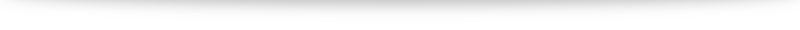# Integration techniques

Discussion (with examples) on integration techniques is complete! (As usual, I welcome comments and info about typos, dead links, etc.)

1. Equations and inequalities
2. Curves and transformations
3. Functions
4. Arithmetic and geometric progressions
5. Series and the sigma notation
6. Differentiation
7. Maclaurin series and binomial expansion
8. Integration techniques
9. Definite integrals
10. Differential equations
11. Vectors
12. Complex numbers

Next up: randomly generated question on integration to test your concepts. Then vectors, which will likely take a whole chunk of time and effort. Let’s work hard together!